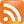Math in the Best of the Web Blog Directory

# Math

#### Blog Directory/Science/Math

Musings from an Australian computational mathematician with a PhD in computer science.

The Algorithmist

Applied math with a focus on computational geometry for Actionscript programmersBlog for and written by grad students.

Annoying Precision

Mathematics undergraduate blogs about the application of probabilistic ideas to prove non-probabilistic facts.The Art of Problem Solving

Questions, lectures and information on mathematical problems and solutions.Climbing Mount Bourbaki

Combinatorics and More

Professor at the Institute of Mathematics at the Hebrew University of Jerusalem blogs about combinatorics and related matters.The Complex Event Processing Blog

Blog highlights news and assessments about the Complex Event Processing (CEP) Community.Computational Complexity

Contains comments, information and notes on mathematical computations.Concrete Nonsense

Presents a group blog about mathematics.

dy/dan

A former high school math teacher writes about math education.

The Endeavour

Blogger with experience as a math professor, programmer, consultant, manager, and statistician blogs about problem solving.GeoGebra Applet Central

Provides free and downloadable GeoGebra applets for students and teachers.God Plays Dice

Offering musings on probability, combinatorics, puzzles, and financial math.The stated mission of this blog is to try to find the fun in "good math".Gowers's Weblog

Offers posts related to mathematics.

Hooda Math Blog

Math teacher Michael Edlavitch uses Flash arcade games, with accompanying worksheets, to teach math.Kitchen Table Math: The Sequel

A group of concerned parents blogging about the state of math education and the US public education system in general.

Malibu Einstein

Mariel writes about math and science.

Math Art

Features arts that were formed by mathematical imagery, and mathematical imagery formed in arts.The Math Forum

Collection of math problems, course plans, links to math resources, and current math news and events.

Math Forum Blogs

Offers a collection of blogs about math from Drexel University.

Math Goes Pop!

Ruminations on the intersection between popular culture and mathematics.Math Overflow

Mathematical Musings

A place for informal mathematical writings: expository, puzzles, art, and open problems, by Matthew Kahle.MathPuzzle

Frequently updated collection of visual math problems.

Maths Is Interesting!

Presents the fun side of maths learning. It also gives pointers to make maths studies smoother and fun.Motivic Stuff

Discussion and links related to the topics of homotopy theory and arithmetic geometry.

Nathaniel Johnston

Featuring recreational mathematics and programming from a Canadian Ph.D. mathematician.The n-Category Café

Offers a group blog on philosophy, math, and physics.

Origami Tessellations

Sentiments and notes on origami, tessellations, geometry, design, and art.Pre-Calculus

Former students of teacher, Mr. Radcliffe offers daily entry tasks, notes, extra homework and answers, and related subject material.Question of the day

Regular postings of math riddles, puzzles and statitical problems.Science and Math Defeated

Dedicated to the critical exploration of contemporary science and mathematics.Secret Blogging Seminar

A group blog by eight Berkeley mathematics Ph.D.'s. Commentary on their research, and other mathematics pursuits.Tanya Khovanova's Math Blog

Blog about mathematics, applications of mathematics to life in general, and the authors life as a mathematician.The Unapologetic Mathematician

Discusses complex mathematical problems in a way which, the author hopes, is accessible to the interested outsider.Vedic Maths Forum India

Features video clips, instruction and tips for using high speed vedic math techniques and tricks.What's New

Updates on the authors research and expository papers, discussion of open problems, and other maths-related topics by Terence Tao.XOR's Hammer

Blogger has a Ph.D in mathematics and shares things in mathematical logic he finds interesting.